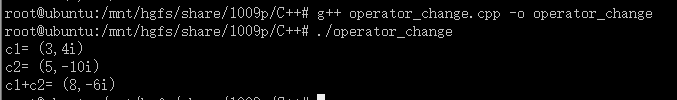• 我们为什么要重载运算符？ 理由就是，我们C++语言中已经给出的运算符（包括算数运算符和逻辑运算符）只是针对C++语言中已经给定的数据类型进行运算，假如我们想要对我们的自定义数据类型进行运算的话，则需要重载...
我们为什么要重载运算符呢？
理由就是，我们C++语言中已经给出的运算符（包括算数运算符和逻辑运算符）只是针对C++语言中已经给定的数据类型进行运算，假如我们想要对我们的自定义数据类型进行运算的话，则需要重载运算符，我们可以把重载运算符理解成对已有的运算符的一种重新定义。
运算符重载有两种方式：重载为类的成员函数&重载为类的友元函数
1成员函数重载运算符
成员函数重载运算符的原型在类的内部
格式如下：
class 类名{
...
返回类型 operator 运算符(形参表)
//函数体
};

在类外定义成员运算符函数的格式为：
返回类型 类名::operator 运算符(形参表){
//函数体
} 
运算符可以分为单目运算符和双目运算符。
1.1单目运算符
用成员函数重载运算符时，如果运算符是单目的，则参数表为空。因为这时操作数访问该重载运算符的对象本身的数据，该对象有this指针指向，所以参数表为空。
struct ti{
int a,b;
bool operator < (ti &y){
if(a !=y.a )return a <y.a ;
return b >y.b ;
}


重载双目运算符时，左边操作数是访问该重载运算符的对象本身的数据，有this指针指向，右边操作数通过成员运算符函数的参数指出。所以，此时成员运算符函数只有一个参数。
 ti operator +(ti &x){
ti ans;
ans.a =a+x.a ;
ans.b =b+x.b ;
return ans;
}
1.3重载++，–运算符
现在C++能识别“++”，“–”运算符是前缀还是后缀的，他们是单目运算符。
类名 operator++（）//前缀方式
类名 operator++（int）//后缀方式。“–”同理
ti operator++(){
++a;
++b;
return *this;
}
ti operator ++(int){
ti temp=*this;
++a;
++b;
return temp;
}
2友元函数重载运算符
用友元函数重载运算符时，因为友元运算符函数没有this指针，所以如果运算符时单目的，则参数表中有一个操作数，如果运算符时双目的，则参数表中有两个操作数。
格式如下：
friend <函数类型> operator <运算符> (<形参>)
{...}
friend <函数类型> operator <运算符> (<形参1>,<形参2>)
{...} 
在重载输出输入运算符的时候，只能采用全局函数的方式（因为我们不能在ostream和istream类中编写成员函数），这里才是友元函数真正的应用场景
 friend istream &operator>>(istream &in,ti &x){
in>>x.a>>x.b;
return in;
}
friend ostream &operator <<(ostream &out,ti &x){
out<<x.a<<" "<<x.b;
return out;
}


展开全文• 以友元函数作为重载运算符的方式重载运算符+ 下面的例子来自于课本 #include using namespace std; class Complex { public: Complex() { real = 0; imag = 0; } Complex(double
以友元函数作为重载运算符的方式重载运算符+
下面的例子来自于课本
#include <iostream>
using namespace std;

class Complex
{
public:
Complex()
{
real = 0;
imag = 0;
}
Complex(double r, double i)
{
real  = r;
imag = i;
}
friend Complex operator + (Complex &c1, Complex &c2); //声明重载运算符
void display();

private:
double real;
double imag;
};

Complex operator +(Complex &c1,Complex &c2)
{
return Complex(c1.real + c2.real, c1.imag + c2.imag);
}

void Complex::display()
{
cout<<"("<<real<<","<<imag<<"i)"<<endl; //输出复数形式
}

int main()
{
Complex c1(3,4), c2(5,-10), c3;
c3 = c1 + c2; //运算符+ 用于复数运算

cout<<"c1= ";
c1.display();

cout<<"c2= ";
c2.display();

cout<<"c1+c2= ";
c3.display();

return 0;
}

此主函数中执行c3 = c1 + c2调用了重载运算符operator +;
并且此重载运算符函数是Complex的友元函数。
必须是Complex对象相加才可以。
运行结果:读者可以自行对比一下友元函数为重载运算符函数和成员函数作为重载运算符函数的区别：http://blog.csdn.net/z_dream_st/article/details/78044481


展开全文C++
• C++学习笔记之运算符重载（赋值运算符，关系运算符重载）C++运算符重载 运算符重载 重载运算符 赋值运算符重载 C++编译器至少要给一个类添加4个函数 默认构造函数 默认析构函数 默认拷贝构造函数 赋值运算符 ...
C++学习笔记之运算符重载（赋值运算符，关系运算符重载）C++运算符重载 运算符重载 重载运算符
赋值运算符重载
C++编译器至少要给一个类添加4个函数

默认构造函数
默认析构函数
默认拷贝构造函数
赋值运算符 operator=对属性进行值拷贝
如果类中有属性指向堆区，做赋值操作的时候会出现深浅拷贝的问题
应该要先判断是否有属性在堆区，如果要先释放干净，然后再进行深拷贝

if(m_Age != NULL){
delete m_Age;
m_Age = NULL;
}

#include <iostream>
using namespace std;

//C++编译器至少给一个类添加4个函数
//1.默认构造函数（无参，函数体为空）
//2.默认析构函数（无参，函数体为空）
//3.默认拷贝构造函数，对属性进行值拷贝
//4.赋值运算符operator=， 对属性进行值拷贝

//如果类中有属性指向堆区，做赋值操作时也会出现深浅拷贝问题

class Person
{
public:

Person(int age)
{
//将年龄数据开辟到堆区
m_Age = new int(age);
}

//重载赋值运算符
Person& operator=(Person& p)
{
if (m_Age != NULL)
{
delete m_Age;
m_Age = NULL;
}
//编译器提供的代码是浅拷贝
//m_Age = p.m_Age;

//提供深拷贝 解决浅拷贝的问题
m_Age = new int(*p.m_Age);

//返回自身
return *this;
}

~Person()
{
if (m_Age != NULL)
{
delete m_Age;
m_Age = NULL;
}
}

//年龄的指针
int* m_Age;

};

void test01()
{
Person p1(18);

Person p2(20);

Person p3(30);

p3 = p2 = p1; //赋值操作

cout << "p1的年龄为：" << *p1.m_Age << endl;

cout << "p2的年龄为：" << *p2.m_Age << endl;

cout << "p3的年龄为：" << *p3.m_Age << endl;
}

int main() {

test01();

//int a = 10;
//int b = 20;
//int c = 30;

//c = b = a;
//cout << "a = " << a << endl;
//cout << "b = " << b << endl;
//cout << "c = " << c << endl;

system("pause");

return 0;
}

关系运算符重载
作用： 重载关系运算符，可以让两个自定义对象进行对比操作
函数调用运算符重载
由于重载之后使用的方法非常像函数的调用，因此称为仿函数
仿函数没有固定写法，非常灵活。
//函数调用运算符（）也可以重载
//由于重载后使用的方式非常像函数的调用，因此称为仿函数
//仿函数没有固定写法，非常灵活
#include <iostream>
#include <string>
using namespace std;
class Person {
//默认构造函数
//默认析构函数
//默认拷贝构造函数
//赋值运算符重载
public:
void operator() (string text){
cout << text << endl;
}
};
void test00() {
//重载的()操作符，也称为仿函数
Person xiaoming;
xiaoming("大家好，我是小明");

}

public:
int operator()(int val1, int val2) {
return val1 + val2;
}
};
void test90() {
cout << "ret = " << ret << endl;
//构建匿名对象
cout << Myadd()(20, 20) << endl;
}
int main() {
test00();
test90();
return 0;
}



展开全文c++
• C++重载运算符和重载函数C++ 允许在同一作用域中的某个函数和运算符指定多个定义，分别称为函数重载和运算符重载。重载声明是指一个与之前已经在该作用域内声明过的函数或方法具有相同名称的声明，但是它们的参数...


C++ 重载运算符和重载函数C++ 允许在同一作用域中的某个函数和运算符指定多个定义，分别称为函数重载和运算符重载。重载声明是指一个与之前已经在该作用域内声明过的函数或方法具有相同名称的声明，但是它们的参数列表和定义(实现)不相同。当您调用一个重载函数或重载运算符时，编译器通过把您所使用的参数类型与定义中的参数类型进行比较，决定选用最合适的定义。选择最合适的重载函数或重载运算符的过程，称为重载决策。C++ 中的函数重载在同一个作用域内，可以声明几个功能类似的同名函数，但是这些同名函数的形式参数(指参数的个数、类型或者顺序)必须不同。您不能仅通过返回类型的不同来重载函数。下面的实例中，同名函数 print() 被用于输出不同的数据类型：#include using namespace std;class printData{public:void print(int i) {cout << "Printing int: " << i << endl;      }void print(double  f) {cout << "Printing float: " << f << endl;      }void print(char* c) {cout << "Printing character: " << c << endl;      }};int main(void){   printData pd;// Call print to print integer   pd.print(5);// Call print to print float   pd.print(500.263);// Call print to print character   pd.print("Hello C++");return 0;}当上面的代码被编译和执行时，它会产生下列结果：Printing int: 5Printing float: 500.263Printing character: Hello C++C++ 中的运算符重载您可以重定义或重载大部分 C++ 内置的运算符。这样，您就能使用自定义类型的运算符。重载的运算符是带有特殊名称的函数，函数名是由关键字 operator 和其后要重载的运算符符号构成的。与其他函数一样，重载运算符有一个返回类型和一个参数列表。Box operator+(const Box&);声明加法运算符用于把两个 Box 对象相加，返回最终的 Box 对象。大多数的重载运算符可被定义为普通的非成员函数或这被定义为类成员函数。如果我们定义上面的函数为类的非成员函数，那么我们需要为每次操作传递两个参数，如下所示：Box operator+(const Box&, const Box&);下面的实例使用成员函数演示了运算符重载的概念。在这里，对象作为参数进行传递，对象的属性使用 this 运算符进行访问，如下所示：#include using namespace std;class Box{public:double getVolume(void){return length * breadth * height;      }void setLength( double len ){          length = len;      }void setBreadth( double bre ){          breadth = bre;      }void setHeight( double hei ){          height = hei;      }// 重载 + 运算符，用于把两个 Box 对象相加      Box operator+(const Box& b)      {         Box box;         box.length = this->length + b.length;         box.breadth = this->breadth + b.breadth;         box.height = this->height + b.height;return box;      }private:double length;      // 长度double breadth;     // 宽度double height;      // 高度};// 程序的主函数int main( ){   Box Box1;                // 声明 Box1，类型为 Box   Box Box2;                // 声明 Box2，类型为 Box   Box Box3;                // 声明 Box3，类型为 Boxdouble volume = 0.0;     // 把体积存储在该变量中// Box1 详述   Box1.setLength(6.0);   Box1.setBreadth(7.0);   Box1.setHeight(5.0);// Box2 详述   Box2.setLength(12.0);   Box2.setBreadth(13.0);   Box2.setHeight(10.0);// Box1 的体积   volume = Box1.getVolume();cout << "Volume of Box1 : " << volume <<endl;// Box2 的体积   volume = Box2.getVolume();cout << "Volume of Box2 : " << volume <<endl;// 把两个对象相加，得到 Box3   Box3 = Box1 + Box2;// Box3 的体积   volume = Box3.getVolume();cout << "Volume of Box3 : " << volume <<endl;return 0;}当上面的代码被编译和执行时，它会产生下列结果：Volume of Box1 : 210Volume of Box2 : 1560Volume of Box3 : 5400可重载运算符/不可重载运算符下面是可重载的运算符列表：+-*/%^&|~!,=<><=>=++--<<>>==!=&&||+=-=/=%=^=&=|=*=<<=>>=[]()->->*newnew []deletedelete []下面是不可重载的运算符列表：::.*.?:运算符重载实例下面提供了各种运算符重载的实例，帮助您更好地理解重载的概念。序号运算符和实例1一元运算符重载2二元运算符重载3关系运算符重载4输入/输出运算符重载5++ 和 -- 运算符重载6赋值运算符重载7函数调用运算符 () 重载8下标运算符 [] 重载9类成员访问运算符 -> 重载展开全文函数已声明不可访问
• 不能被重载的运算符 1、. (成员访问运算符) 2、.* (成员指针访问运算符) 3、:: (域运算符) 4、sizeof(长度运算符) 5、?: (条件运算符） 重载运算符
• 重载运算符 您可以重定义或重载大部分 C++ 内置的运算符。这样，您就能使用自定义类型的运算符。 重载的运算符是带有特殊名称的函数，函数名是由关键字 operator 和其后要重载的运算符符号构成的。 与其他函数一样，...
• 用运算符重载函数作为类成员函数的方法重载运算符+ 下面的例子来自于课本： #include using namespace std; class Complex { public: Complex() //无参构造函数 { real = 0; imag = 0; } CoC++ 函数
• 重载运算符/不可重载运算符下面是可重载的运算符列表：双目算术运算符+ (加)，-(减)，*(乘)，/(除)，% (取模)关系运算符==(等于)，!= (不等于)，&lt; (小于)，&gt; (大于&gt;，&lt;=(小于等于)，...
• 1.对双目运算符而言，成员函数重载运算符的函数参数表中只有一个参数，而用友元函数重载运算符函数参数表中含有两个参数。  对单木运算符来说，成员函数重载运算符的函数参数表中没有参数，而用友元函数重载运算符...
• 利用C++实现Point类重载运算符。利用C++实现Point类重载运算符
• 本文较为详细的描述了重载运算符的方法。一般来说，重载运算符在实际的项目开发中会经常的用到，但如果某些自定义类型通过简短几行代码重载一些常用的运算符（如：+-*/），就能让编程工作带来方便；重载运算符就是......